# How to Use rowMeans() Function in R

R rowMeans() function is used to calculate the mean of each row of a data frame or matrix.

## Syntax

``rowMeans(x, na.rm = FALSE)``

## Parameters

1. x: It is an array of two or more dimensions containing numeric, complex, integer, or logical values or a numeric data frame.
2. na.rm: It is a logical argument. If TRUE, NA values are ignored.

## Example 1: Calculating the mean of every row of a data frame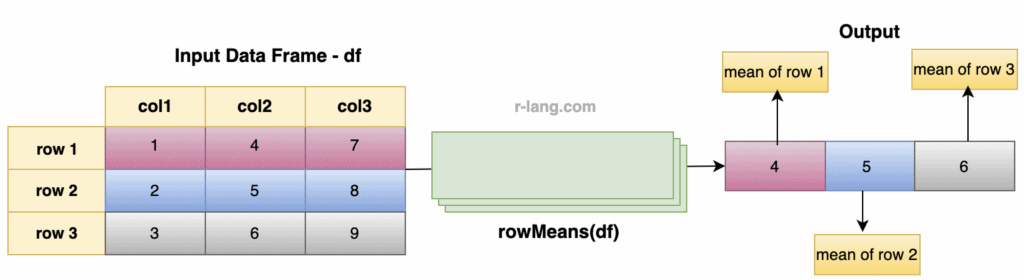``````# Create data frame
df <- data.frame(
player1 = c(11, 55, 99, 444, 888),
player2 = c(22, 66, 111, 555, 999),
player3 = c(33, 77, 222, 666, 11),
player4 = c(44, 88, 333, 777, 22)
)

# Calculating the mean of every row using rowMeans() function
rowMeans(df)
``````

Output

`````` 27.50  71.50  191.25  610.50  480.00
``````

We calculated the mean of the values across each row using the rowMeans() function, effectively providing the average value for each player across the columns.

## Example 2: Calculating the mean of every row and excluding NA values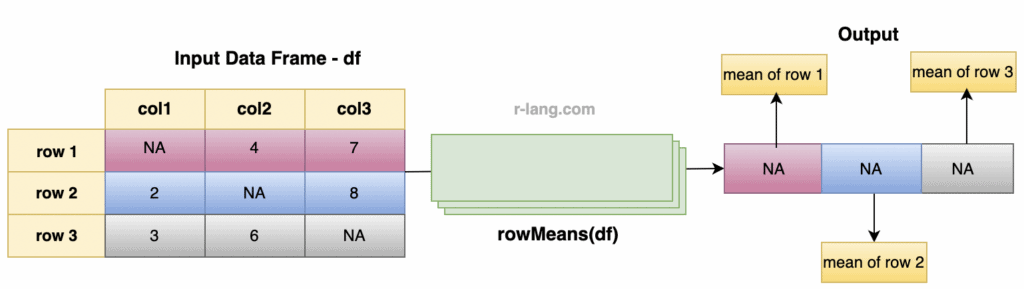As you can see from the figure, we get the output NA for every row because every row contains NA values.

``````# Create a data frame.
df <- data.frame(
col1 = c(NA, 2, 3),
col2 = c(4, NA, 6),
col3 = c(7, 8, NA)
)

# Calculate the mean of data frame
rowMeans(df)
``````

Output

`````` NA NA NA
``````

You can exclude NA values while calculating the mean of every row by passing the na.rm = TRUE parameter to the rowMeans() function.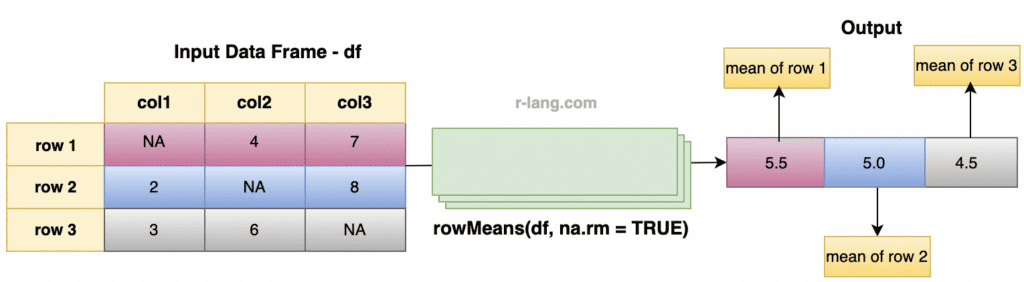``````# Create a data frame.
df <- data.frame(
col1 = c(NA, 2, 3),
col2 = c(4, NA, 6),
col3 = c(7, 8, NA)
)

# Calculate the mean of data frame
rowMeans(df, na.rm = TRUE) ``````

Output

`````` 5.5   5.0   4.5
``````

## Example 3: Calculating the mean of specific rows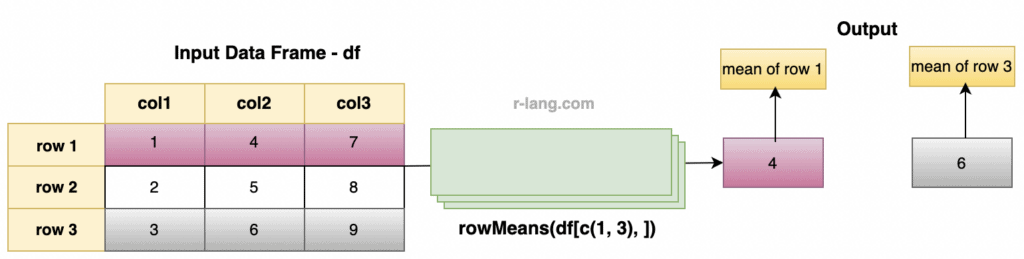``````# Create a data frame.
df <- data.frame(
col1 = c(1, 2, 3),
col2 = c(4, 5, 6),
col3 = c(7, 8, 9)
)
rowMeans(df[c(1, 3), ])``````

Output

``````  1   3
4   6
``````

From the output and figure, you can see that for row 1, the mean is 4, and for row 2, the mean is 6.

## Example 4: Calculating the mean of every row of the matrix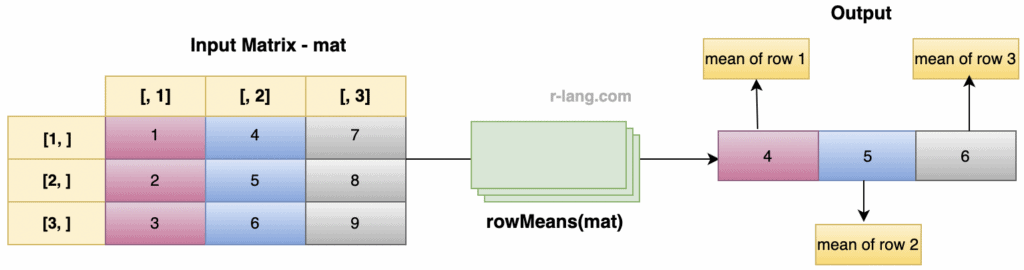``````# Create a matrix.
mat <- matrix(1:9, 3, 3)
print(mat)

# Calculate the mean of each row.
cat("The mean of every row of matrix: ", rowMeans(mat), "\n")
``````

Output

``````The mean of every row of matrix: 4  5  6
``````